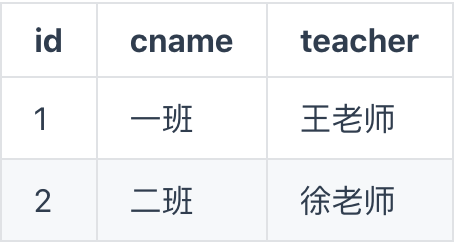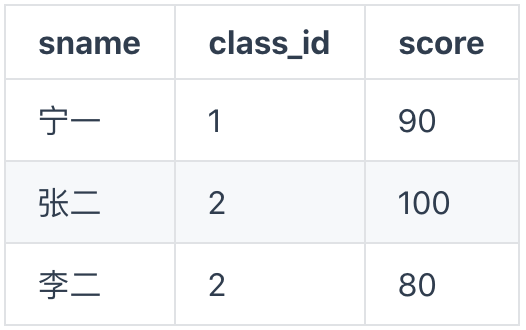# 置顶最佳实践丨云数据库实现联表+聚合查询精选热门

### 场景说明

class（班级信息）：student（学生信息）：## 代码示例

### 1、lookup 联表查询

```lookup({
from: "student", //要关联的表student
localField: "id", //class表中的关联字段
foreignField: "class_id", //student表中关联字段
as: "stu" //定义输出数组的别名
}).end();
```

```{"list":
[{
"id":1,
"teacher":"王老师",
"cname":"一班",
"stu":[
{
"sname":"宁一",
"class_id":1,
"score":90
}
]
},
{
"id":2,
"teacher":"徐老师",
"cname":"二班",
"stu":[
{
"class_id":2,
"sname":"张二",
"score":100
},
{
"class_id":2,
"sname":"李二",
"score":80
}
]
}]
}
```

### 2、match 条件匹配

```.lookup({
from: 'student',
localField: 'id',
foreignField: 'class_id',
as: 'stu'
})
.match({
teacher:"徐老师"
})
.end()
```

```{
"list": [
{
"_id": "5e847ab25eb9428600a512352fa6c7c4",
"id": 2,
"teacher": "徐老师",
"cname": "二班",
//学生数据
"stu": [
{ "_id": "37e26adb5eb945a70084351e57f6d717", "class_id": 2, "sname": "张二", "score": 100 },
{ "_id": "5e847ab25eb945cf00a5884204297ed8", "class_id": 2, "sname": "李二", "score": 80 }
]
}
]
}
```

### 3、直接返回学生成绩平均值

```.lookup({
from: 'student',
pipeline: \$.pipeline()
.group({
_id: null,
score: \$.avg('\$score')
})
.done(),
as: 'stu'
})
.match({
teacher:"徐老师"
})
.end()
```

```{
"list": [
{
"_id": "5e847ab25eb9428600a512352fa6c7c4",
"id": 2,
"teacher": "徐老师",
"cname": "二班",
"stu": [{ "_id": null, "score": 90 }]
}
]
}
```

### 4. 只显示 teacher 和 score 这两个值

```.lookup({
from: 'student',
pipeline: \$.pipeline()
.group({
_id: null,
score: \$.avg('\$score')
})
.done(),
as: 'stu'
})
.match({
teacher:"徐老师"
})
.replaceRoot({
newRoot: \$.mergeObjects([\$.arrayElemAt(['\$stu', 0]), '\$\$ROOT'])
})
.project({
_id:0,
teacher:1,
score:1
})
.end()
```

```{ "list": [{ "score": 90, "teacher": "徐老师" }] }
```

## 产品介绍